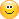# New candle714

Anyone got a good piece of code for build 600+ that will determine if a tick that came in is the start of a new candle or not? The code I have doesn't work anymore.Moderator
19103

nondisclosure:
Anyone got a good piece of code for build 600+ that will determine if a tick that came in is the start of a new candle or not? The code I have doesn't work anymore.

Show the code that you have. I can't think of any reason why it would not work if it worked before714

Here it is. The other problem it has is it's based on time. I'm using Renko charts, so I need a way that doesn't rely on time. PS. This code doesn't work either. No errors, it just misses the candle. I snargled this from an mql4.com forum post years ago.

```bool funcIsNewBar(int timeFrame)
{
bool res=false;

// the array contains open time of the current (zero) bar
// for 7 (seven) timeframes
static datetime _sTime;
int i=6;
//Note: i below will be 6 or timeframe will be day.
switch (timeFrame)
{
case 1  : i=0; break;
case 5  : i=2; break;
case 15 : i=3; break;
case 30 : i=4; break;
case 60 : i=5; break;
case 240: break;
case 1440:break;
default:  timeFrame = 1440;
}
//----
if (_sTime[i]==0 || _sTime[i]!=iTime(Symbol(),timeFrame,0))
{
_sTime[i] = iTime(Symbol(),timeFrame,0);
res=true;
}

//----
return(res);
}
```19999

GumRai:

nondisclosure:
Anyone got a good piece of code for build 600+ that will determine if a tick that came in is the start of a new candle or not? The code I have doesn't work anymore.
Show the code that you have. I can't think of any reason why it would not work if it worked before
 I agree, if it was good before, it should still be. ```void OnTick(){    static datetime timeCur; datetime timePre = timeCur; timeCur=Time;    bool isNewBar = timeCur != timePre;    if(isNewBar){      : // Once per bar    }    : // every tick }``` Note the above does not handle chart changes714

WHRoeder:
 I agree, if it was good before, it should still be. Note the above does not handle chart changes

Hey WHRoeder, been meaning to ask:

This may be the stroke talking but does this line of code :

`bool isNewBar = timeCur != timePre;`

mean the same same thing as these lines of code:

```if (timeCur != timePre) isNewBar=true;
else isNewBar=false;```714

nondisclosure:

Hey WHRoeder, been meaning to ask:

This may be the stroke talking but does this line of code :

mean the same same thing as these lines of code:

New problem with the code. When the first tick comes in after the code is launched, it thinks there's a new candle even though there isn't.3196

nondisclosure:
New problem with the code. When the first tick comes in after the code is launched, it thinks there's a new candle even though there isn't.

then make it happen exactly the way you want it

```void OnTick(){
static datetime timeCur = Time; datetime timePre = timeCur; timeCur=Time;
bool isNewBar = timeCur != timePre;
if(isNewBar){
: // Once per bar
}
: // every tick
}```19999

1. nondisclosure:

his may be the stroke talking but does this line of code :

`bool isNewBar = timeCur != timePre;`

mean the same same thing as these lines of code:

```if (timeCur != timePre) isNewBar=true;
else isNewBar=false;```
 ```if (timeCur != timePre) isNewBar=true; else                    isNewBar=false;``` ```if (condition) isNewBar=condition; else           isNewBar=condition;``` `isNewBar=timeCur != timePre;` `isNewBar=condition;`
2. qjol: then make it happen exactly the way you want it
`   static datetime timeCur = Time; datetime timePre = timeCur; timeCur=Time;`
This won't compile. Must be initialized with a constant.

3. nondisclosure: New problem with the code. When the first tick comes in after the code is launched, it thinks there's a new candle even though there isn't.
You have to code it properly.
```datetime time0; int init(){ time0 = Time;  // No mid bar allowed.
:
}
int start(){
bool isNewBar = time0 != Time; time0 = Time;```
4. or
```datetime time0; int init(){
time0 = Time;                           // No mid bar allowed unless it's
if(TimeCurrent()-time0 < _Period) time0=0; // less than 1.7% of a new candle.
:
}
int start(){
bool isNewBar = time0 != Time; time0 = Time; ```38121

WHRoeder:

1. qjol: then make it happen exactly the way you want it
`static datetime timeCur = Time;`
This won't compile. Must be initialized with a constant.19999

1. I missed the other post.
2. It shouldn't compile, non-constant expression.
 Static Variables - MQL4 Documentation A static variable can be initialized by a constant or constant expression corresponding to its type, unlike a simple local variable, which can be initialized by any expression. Initialization of Variables - MQL4 Documentation Global and static variables can be initialized only by a constant of the corresponding type or a constant expression. Local variables can be initialized by any expression, not just a constant.38121

WHRoeder:
1. I missed the other post.
2. It shouldn't compile, non-constant expression.
 Static Variables - MQL4 Documentation A static variable can be initialized by a constant or constant expression corresponding to its type, unlike a simple local variable, which can be initialized by any expression. Initialization of Variables - MQL4 Documentation Global and static variables can be initialized only by a constant of the corresponding type or a constant expression. Local variables can be initialized by any expression, not just a constant.

I knowIt seems Predefined Variables are special cases.

Values of predefined variables are set by the client terminal before a mql4-program is started. Predefined variables are constant and cannot be changed from a mql4-program.